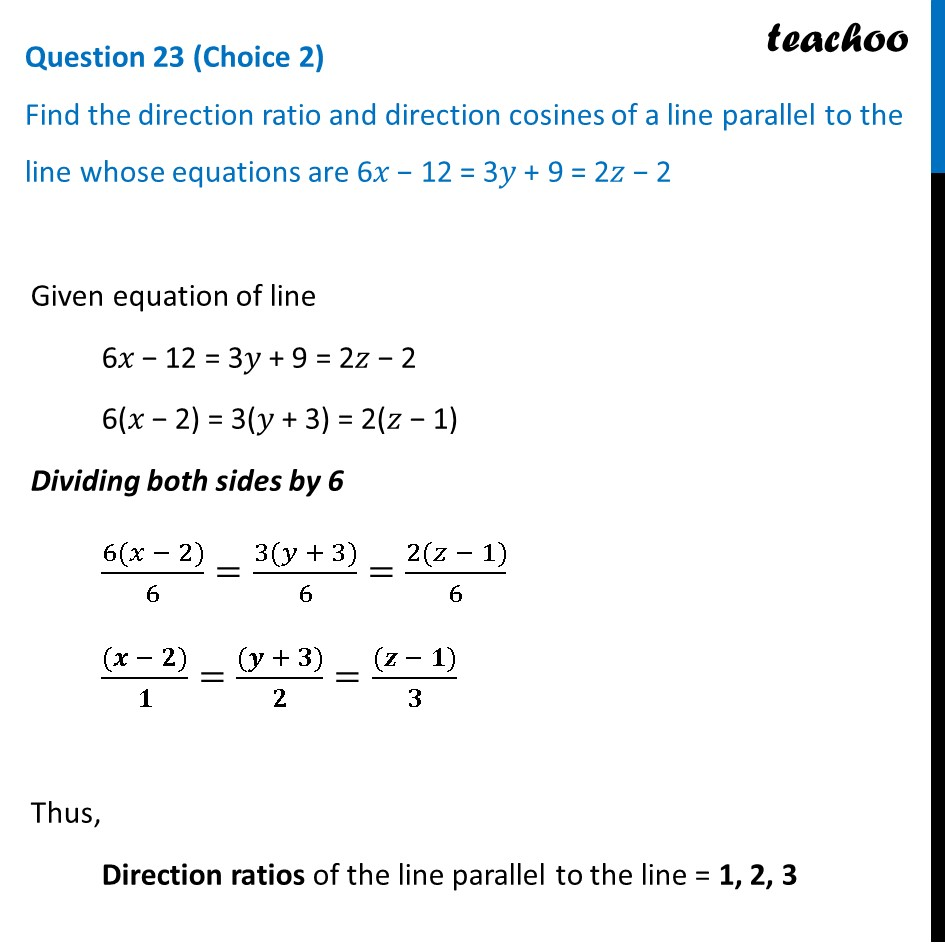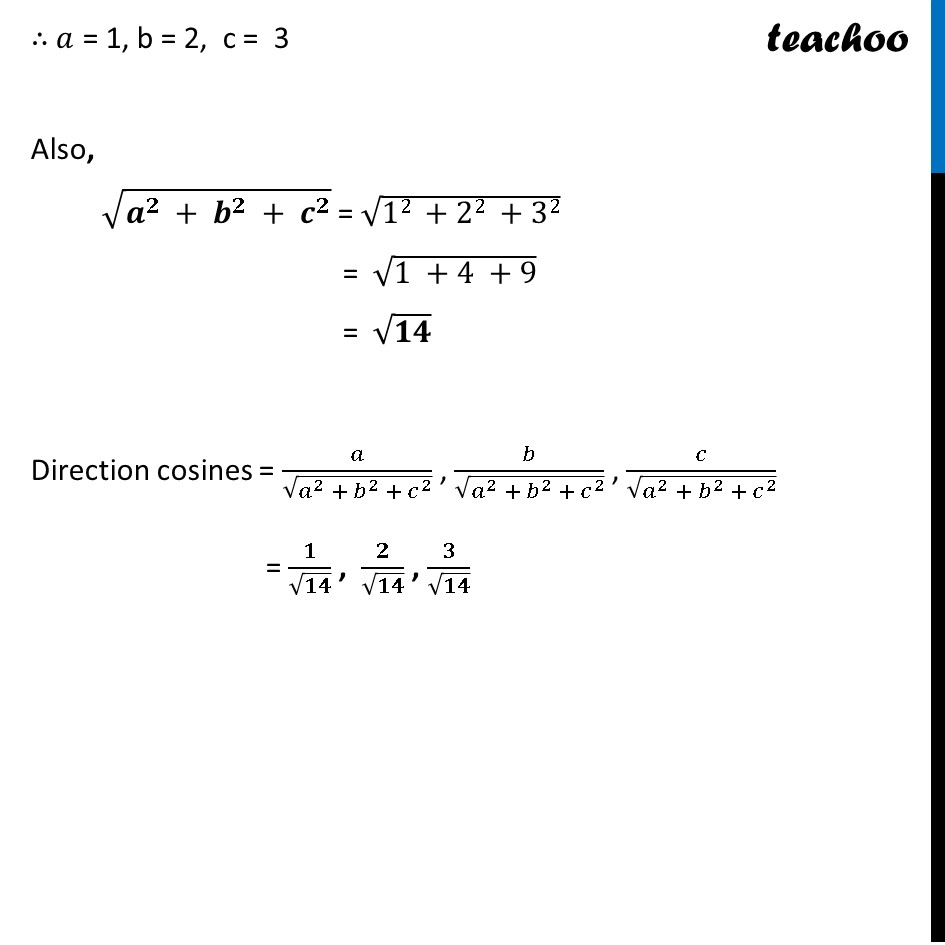CBSE Class 12 Sample Paper for 2023 Boards

Class 12
Solutions of Sample Papers and Past Year Papers - for Class 12 Boards

##googletag.cmd.push(function() { googletag.display('div-gpt-ad-1669298377854-0'); });(adsbygoogle = window.adsbygoogle || []).push({});

Learn in your speed, with individual attention - Teachoo Maths 1-on-1 Class

### Transcript

Question 23 (Choice 2) Find the direction ratio and direction cosines of a line parallel to the line whose equations are 6𝑥 − 12 = 3𝑦 + 9 = 2𝑧 − 2 Given equation of line 6𝑥 − 12 = 3𝑦 + 9 = 2𝑧 − 2 6(𝑥 − 2) = 3(𝑦 + 3) = 2(𝑧 − 1) Dividing both sides by 6 (6(𝑥 − 2))/6=(3(𝑦 + 3))/6=(2(𝑧 − 1))/6 ((𝒙 − 𝟐))/𝟏=((𝒚 + 𝟑))/𝟐=((𝒛 − 𝟏))/𝟑 Thus, Direction ratios of the line parallel to the line = 1, 2, 3 ∴ 𝑎 = 1, b = 2, c = 3 Also, √(𝒂^𝟐 + 𝒃^𝟐 + 𝒄^𝟐 ) = √(12 +22 +32) = √(1 +4 +9) = √𝟏𝟒 Direction cosines = 𝑎/√(𝑎^2 + 𝑏^2 + 𝑐^2 ) , 𝑏/√(𝑎^2 + 𝑏^2 + 𝑐^2 ) , 𝑐/√(𝑎^2 + 𝑏^2 + 𝑐^2 ) = 𝟏/√𝟏𝟒 , 𝟐/√𝟏𝟒 , 𝟑/√𝟏𝟒